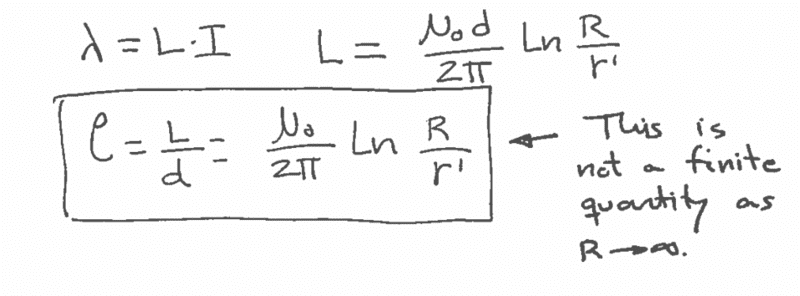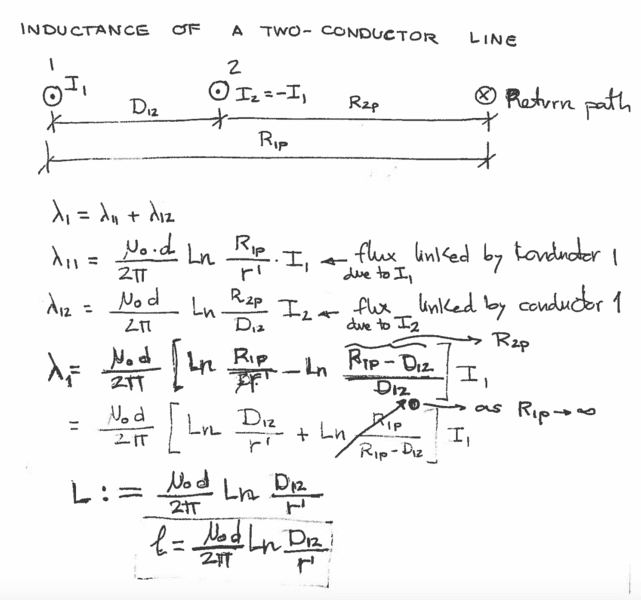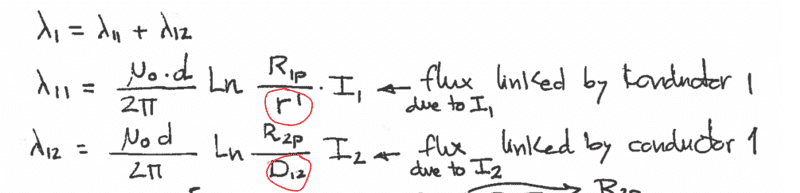# Inductance of two-conductor line - Proof Questions

• Marcin H
GMD.In summary, to derive equations for the inductance of an x-conductor line, you need the equation for the per unit length inductance, which is Ln(r'). The equation for the per unit length inductance is based on the mutual inductance of filaments in a conductor, and it depends on the frequency of the current and the radius of the conductor. To find the GMD of a round conductor, you need to compute the n'th root of the product of the n inputs.f

#### Marcin H

I am trying to understand how to derive equations for the inductance of an x-conductor line. Any number really. But I want to understand the proof for a two-conductor line first. So to start any of these proofs you first need the equation for the per unit length inductance:I know R is the distance between the wire and the return path, but I am confused by what r' is in that formula. I know that r' = e^(1/4)*r, where r is the radius of the wire we are looking at. But what does that term in front of r do? What do we call r' or how do we think about it physically?

Then we get into the proof itself. Here it is from my notes:I can follow this for the most part by applying the first equation, but the thing I don't understand at all is where the denominator is coming from in the Ln part of each equation. Why is the denominator for λ_11 r'? and why is the denominator D_12 for the λ_12? This brings me back to my first question of what is r' and how does it change depending on what wire you are looking at. r' is where I get really confused. (Circled in red below)#### Attachments

Did I post this in the wrong place btw?

Did I post this in the wrong place btw?
I think it is just saying that the inductance increases logarithmically as the ratio of spacing to radius increases. And it never reaches a maximum.

I was confused by your question. You refer to a “two conductor line” or an x-conductor line. That sounds like a transmission line.
But your diagram shows two conductors carrying current in the same direction, with a separate return current path. It is normal to consider multiple paralleled conductors as a “cage”. A cage of conductors has lower inductance than a single wire carrying the whole current.

When used for EHV power transmission the cage reduces corona breakdown because a cage of wires looks electrostaticaly like a larger radius conductor.

Some radio antennas benefit from maintaining the conductor-over-ground impedance by tapering the wire cage. That changes both inductance and capacitance so can correct impedance along the wire that would be distorted by nearby conductive structures such as masts and towers.

The information needed to calculate the inductance of practical paralleled conductors is available, but first it helps to know why you are interested and where you want to take it. There are some fun concepts out there, like the Geometric Mean Distance, GMD, of a circle from itself.

•Fisherman199, dlgoff and anorlunda
So to start any of these proofs you first need the equation for the per unit length inductance:
These are not “proofs”, they are from a suite of excellent numerical approximations that were first identified by a brilliant applied mathematician called J. C. Maxwell.
See; A Treatise on Electricity and Magnetism, Vol 2, Chap XIII, with attention to article 692.
https://ia902302.us.archive.org/25/items/ATreatiseOnElectricityMagnetism-Volume1/Maxwell-ATreatiseOnElectricityMagnetismVolume1_text.pdf
https://ia600304.us.archive.org/9/items/ATreatiseOnElectricityMagnetism-Volume2/Maxwell-ATreatiseOnElectricityMagnetismVolume2_text.pdf

Start by imagining a filamentary current with a position, but with zero radius. That filament has self-inductance, which is the coefficient of magnetic coupling with itself. That filament can share magnetic fields with other filaments, each through a coefficient of mutual-inductance. The sum of all those interactions gives the total inductance of the collection of filaments making a conductor.

Now imagine a round conductor having a radius. That conductor guides a regular distribution of filaments. The inductance of the whole can be modeled, but the approximation selected will depend on the frequency of the current and the radius of the conductor. For DC and low frequencies, a round conductor can be modeled as a round area of equally distributed filaments. For high frequency current, skin effect reduces the model to a surficial tube of filaments.

At each stage in the hierarchical modelling, the mutual interaction of all filaments with all other filaments must be considered to obtain a coefficient for that stage. That is where the concept of the Geometric Mean Distance comes in. It gives the mean coupling of any filament with any other filament being considered. For round conductors at DC and low voltages it is the GMD of a filled circle from itself that is needed. For higher frequencies it is the GMD of only a narrow annulus from itself that is important.

When winding coils from round wire, the coupling of any two turns is proportional to the GMD of two circles. The integral of all turns involves a coefficient based on the GMD of the distribution of the turns on the coil.

To compute a discrete GM requires computation of the n'th root of the product of the n inputs. For many discrete inputs or for continuous areas, integrating or summing the Logs of the inputs, then dividing by the area gives the Log of the GM. You must avoid including the GMD of any filament from itself as that leads to the obvious pitfall at Log(zero).

We see exponential and logarithmic functions throughout inductance computations because the filamentary building blocks used to model our conductors in the real world are all connected conveniently through the world of Maxwell's GMDs.

I think that goes most of the way to explaining why the logarithmic form of equation appears in computing the inductance of geometrically arranged conductors. It also explains the importance of distinguishing between filaments, conductors and arrangements of several parallel conductors.

Professor Andrew Gray did much to rationalise and teach Maxwell's GMD approach.
See; The Theory and Practice of Absolute Measurements in Electricity and Magnetism. Vol 2 (theory ). 1893. Chap VI, Section II, Calculation of Coefficients of Induction. Starts on page 288.
•